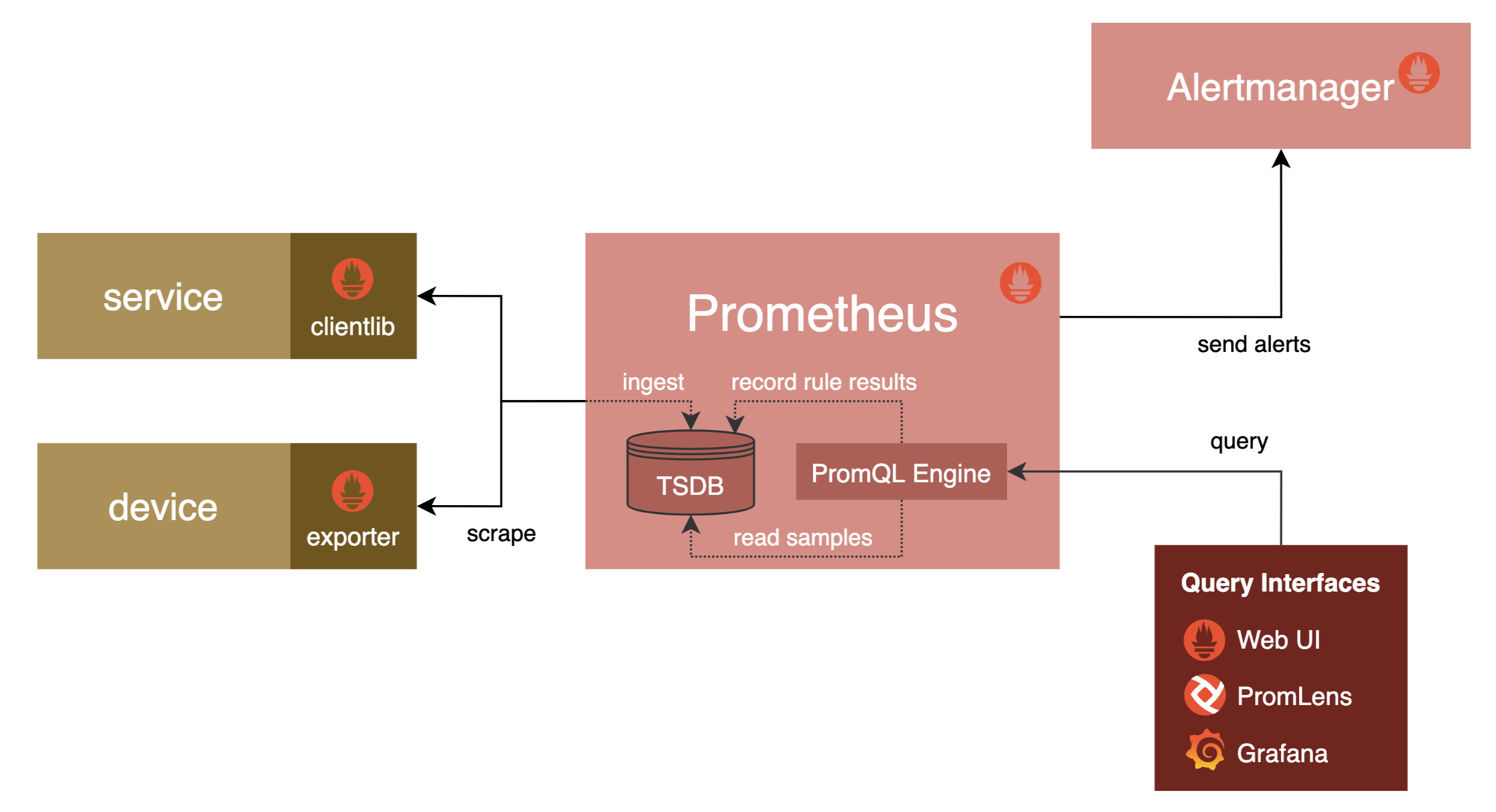# 查询语言¶## 示例¶

``````http_requests_total{status="500"}
``````

``````rate(http_requests_total{status="500"}[5m])
``````

``````sum by(path) (rate(http_requests_total{status="500"}[5m]))
/
sum by(path) (rate(http_requests_total[5m]))
``````

## 集成报警¶

Prometheus 将时间序列数据的收集、处理和主动报警系统集成在一起，它的理念是在单个数据模型中尽可能多地收集有关系统的数据，以便你可以对其进行集成查询。此外用于临时查询和仪表盘使用的查询语言也可以用于定义报警规则。

``````alert: Many500Errors
# expr 是一个 PromQL 表达式，是整个报警规则的核心
expr: |
(
sum by(path) (rate(http_requests_total{status="500"}[5m]))
/
sum by(path) (rate(http_requests_total[5m]))
) * 100 > 5
for: 5m
labels:
severity: "critical"
annotations:
summary: "Many 500 errors for path {{\$labels.path}} ({{\$value}}%)"
``````